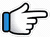# Sach Keh Raha Hai Deewana Dil – Keyboard Notes## Sach Keh Raha Hai Deewana Keyboard Notes

For Sach Keh Raha Hai Deewana Notes as Western or Indian Notations, Click –

Western – CDE FGAB

Indian – srg mpdn

Hindi – सारेग मपधनि

## Keyboard Notes for Sach Keh Raha Hai Deewana – Indian Notation (srg mpdn)

CDE FGAB

सारेग मपधनि

### Part 1

r*         r*      R*R*n   n
Sach   keh   raha      hai

NNnn~        R*~
deewana     dil

r*      r*   R*R*n   n
Dil   na   kisi       se

NNnn
lagaana

r*r*          R*    R*nn   N
Jhoote    hai   yaar     ke

Nnn~    R*M*g*r*

r*r*          R*     R*nn    N
Jhoothi   hai    pyaar   ki

R*nn~
kasmein

g*g*      g*     G*G*g*
Maine   har   lamha

g*G*g*    M*p*g*
jisse        chaaha

g*G*g*    M*p*g*
jisse        pooja

p*p*        M*    M*g*      g*r*    r*R*
Usi          ne     yaaron    mera    dil
Or
M*M*    g*    g*r*           r*R*   R*n

n~R*~    n~ R*~
toda       toda

nnR*~     nnR*M*~   r*R*~
tanha     tanha         chhoda~

Repeat:

r*         r*      R*R*n  n      NNnn~     R*~
Sach   keh   raha      hai   deewana     dil

r*       r*   R*R*n    n   NNnn
Dil   na   kisi         se   lagaana

r*r*          R*    R*nn   N    Nnn~   R*M*g*r*
Jhoote    hai   yaar     ke   wade    saare

r*r*          R*     R*nn   N   R*nn~
Jhoothi   hai   pyaar   ki    kasmein

g*g*       g*     G*G*g*  g*G*g*    M*p*g*
Maine   har   lamha     jisse          chaaha

g*G*g*     M*p*g*
jisse         pooja

p*p*    M*    M*g*      g*r*      r*R*
Usi      ne     yaaron    mera    dil

n~R*~    n~ R*~  nnR*~     nnR*M*~   r*R*~
toda       toda       tanha      tanha        chhoda~

### Part  2

r~ g~         r~ g~
Mausam   mausam

r  gMp   Mg

r~ g~        r~ g~
Mausam   mausam

rg          Mp     Mg
Maine   dekha   usse

DD      D      D R D
hua    main   paagal
Or
DD      D      D r D

DD     nd    Dn      d
bas    pal   bhar   mein
Or
Dd     nd    Dn      d

rRd  pM
AA Aaa

Repeat:

r~ g~         r~ g~
Mausam   mausam

r  gMp   Mg               r~ g~        r~ g~

rg            Mp      Mg     DD    D      D R D
Maine   dekha   usse   hua    main   paagal

DD     nd    Dn      d
bas    pal    bhar   mein

M~ n    M n     r*     R* n
Aake     basi    hai   woh
Or
d~ n      M n    r*   R* n

dd        Dn       d
mere   mann   mein

M~ p    rp       n dp
Uski     kami   hai_ab

M~ gp      M
jeevan      mein

M~      n M n
Woh   door_ hai

r*R*~    ddDnd
meri     nazron_ se
Or
r*R*~   ndDnd

p~       rp        n        nr*R*~  nN~
kyon   usse   main   chaahoon~~

Repeat Full Part 1:

Sach keh raha hai deewana dil…..

Wil be Updated:

Sundar sundar
Main to khone laga uske nashe mein

g*g*      g*     G*G*g*    g*G*g*   M*p*g*
Maine   har   lamha       jisse       chaaha

g*G*g*    M*p*g*
jisse        pooja

p*p*    M*    M*g*      g*r*    r*R*
Usi      ne     yaaron    mera    dil

n~R*~  n~ R*~  nnR*~  nnR*M*~   r*R*~
toda     toda      tanha    tanha         chhoda~

### Sach Keh Raha Hai Deewana Keyboard Notes

r* r* R*R*n n NNnn~ R*~
Sach keh raha hai deewana dil

r* r* R*R*n n NNnn
Dil na kisi se lagaana

r*r* R* R*nn N Nnn~ R*M*g*r*
Jhoote hai yaar ke wade saare

r*r* R* R*nn N R*nn~
Jhoothi hai pyaar ki kasmein

g*g* g* G*G*g*
Maine har lamha

g*G*g* M*p*g*, g*G*g* M*p*g*
jisse chaaha, jisse pooja

p*p* M* M*g* g*r* r*R*
Usi ne yaaron mera dil

n~R*~ n~ R*~, nnR*~ nnR*M*~ r*R*~
toda toda, tanha tanha chhoda~

Repeat:

r* r* R*R*n n NNnn~ R*~
r* r* R*R*n n NNnn
r*r* R* R*nn N Nnn~ R*M*r*R*
r*r* R* R*nn N R*nn~

Sach keh raha hai deewana dil
Dil na kisi se lagaana
Jhoote hai yaar ke wade saare
Jhoothi hai pyaar ki kasmein

g*g* g* G*G*g*
g*G*g* M*p*g* g*G*g* M*p*g*
p*p* M* M*g* g*r* r*R*
n~R*~ n~ R*~ nnR*~ nnR*M*~ r*R*~

Maine har lamha
jisse chaaha jisse pooja
Usi ne yaaron mera dil
toda toda tanha tanha chhoda~

r~ g~ r~ g~
Mausam mausam

r gMp Mg r~ g~ r~ g~

rg Mp Mg
Maine dekha usse

DD D D R D DD nd Dn d
hua main paagal bas pal bhar mein
Or
DD D D r D Dd nd Dn d

rRd pM
AA Aaa

Repeat:

r~ g~ r~ g~
r gMp Mg r~ g~ r~ g~
rg Mp Mg DD D D R D
DD nd Dn d

Mausam mausam
Maine dekha usse hua main paagal
bas pal bhar mein

M~ n M n r* R* n dd Dn d
Aake basi hai woh mere mann mein

M~ p rp n dp M~ gp M
Uski kami hai_ab jeevan mein

M~ n M n r*R*~ ddDnd
Woh door_ hai meri nazron_ se

p~ rp n nr*R*~ nN~
kyon usse main chaahoon~~

Repeat Part 1

Wil be Updated:

Sundar sundar
Main to khone laga uske nashe mein

g*g* g* G*G*g* g*G*g* M*p*g*
g*G*g* M*p*g*
p*p* M* M*g* g*r* r*R*
n~R*~ n~ R*~ nnR*~ nnR*M*~ r*R*~

Maine har lamha jisse chaaha
jisse pooja
Usi ne yaaron mera dil
toda toda tanha tanha chhoda~

Song List

Bhajan List

New Song List